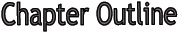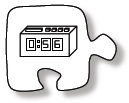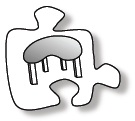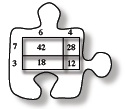# Chapter 2

Chapter 2 Focus

Guiding Questions:

• How can I organize it?
• What information can I get?
• How can I calculate it?
• How can I measure area?
• How do area and perimeter change?

Mathematical Vocabulary

• The following is a list of vocabulary found in this chapter.  Some of the words have been seen in a previous chapter.  The italicized words are new to this chapter.  Make sure that you are familiar with the terms below and know what they mean.  Click on the word for a “pop-up” definition. For more information, refer to the glossary or index.  You might also add these words to your Toolkit so that you can reference them in the future.
•  area bar graph centimeter expression generic rectangle greatest common factor histogram inch length operation perimeter stem-and-leaf plot unit of measure Venn diagram
••Section 2.1 To begin the chapter, you will learn several ways to represent data that you collect.  You will learn to make mathematically justified statements about how accurately your class was able to determine the length of one minute without using a clock!Section 2.2 Section 2.2 focuses on area.  You will learn how to measure area using a variety of units, and why standard units are useful.  You will also learn about perimeter and its relationship to area.Section 2.3 In this section, you will take a closer look at multiplication and how it relates to area.

(taken from CPM CC1 Book)

Chapter Focus – blog entry

Keynotes:

Lesson 2.1.1

Lesson 2.1.2

Lesson 2.2.1

Lesson 2.2.2

Lesson 2.2.3

Lesson 2.3.1

Lesson 2.3.2

Lesson 2.3.3

Lesson 2.3.4